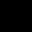# A person’s present age is two-fifth of the age of his mother. After 8 years, he will be one-half of the age of his mother. How old id the mother at present?

## 2 Answers on this Question

1.narmada says:

let us take son age as ‘y’
mom’s age as ‘x’
y is two fifth of x ,then y=(2/5)x____eq1
after 8 years, y+8=(1/2)x_________eq2
subtracting eq2 from eq1,
-8=(2x/5)-(x/2)
x=80
in the question ,they asked current mom’s age ,so 80+8
2.MAMIDI LIJITA SREE says: